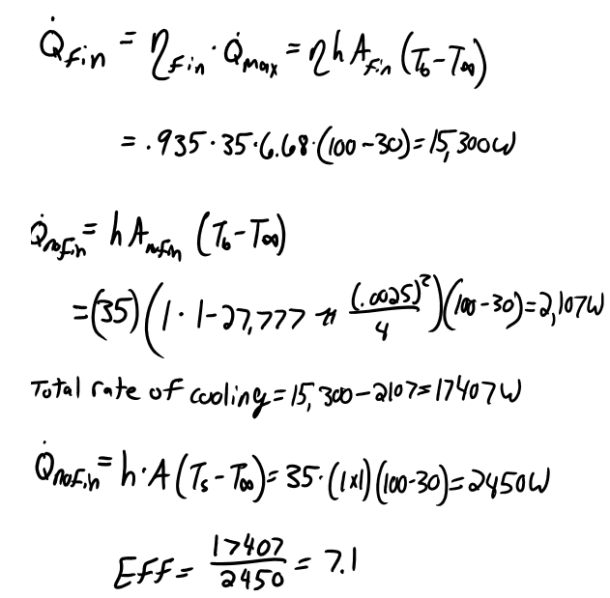heat and mass transfer problems engineering equations heat and mass transfer school homework engineering heat and mass transfer formulas heat and mass transfer solutions to heat and mass transfer problems full solution engineering problem solution heat and mass transfer math problems engineering equations heat and mass transfer school homework engineering solutions to heat and mass transfer formulas heat problems mass problem solutions to transfer problems full solution heat and mass transfer
heat and mass transfer problems engineering equations heat and mass transfer school homework engineering heat and mass transfer formulas heat and mass transfer solutions to heat and mass transfer problems full solution engineering problem solution heat and mass transfer math problems engineering equations heat and mass transfer school homework engineering solutions to heat and mass transfer formulas heat problems mass problem solutions to transfer problems full solution heat and mass transfer
Highalphabet Home Page heat and mass transfer problem solutions Heat and Mass Transfer Page
A hot surface at 100 C is to be cooled by attaching 3-cm-long, 0.25-cm-diameter aluminum pin fins (k=237 W/mK) to it, with a center-to-center distance of 0.6 cm. The temperature of the surrounding medium is 30 C, and the heat transfer coefficient on the surfaces is 35 W/m^2 K. Determine the rate of heat transfer from the surface for a 1-m x 1-m section of the plate. Also determine the overall effectiveness of the fins.A hot surface at 100 C is to be cooled by attaching 3-cm-long, 0.25-cm-diameter aluminum pin fins (k=237 W/mK) to it, with a center-to-center distance of 0.6 cm. The temperature of the surrounding medium is 30 C, and the heat transfer coefficient on the surfaces is 35 W/m^2 K. Determine the rate of heat transfer from the surface for a 1-m x 1-m section of the plate. Also determine the overall effectiveness of the fins.